Courses

# Short Answers - Motion, Science, Class 9 Class 9 Notes | EduRev

## Class 9 : Short Answers - Motion, Science, Class 9 Class 9 Notes | EduRev

The document Short Answers - Motion, Science, Class 9 Class 9 Notes | EduRev is a part of the Class 9 Course Class 9 Science by VP Classes.
All you need of Class 9 at this link: Class 9

Q1. Give the difference between distance and displacement.
Ans.

 Distance Displacement 1. It is the total path covered by an object. 1. It is the shortest path measured from initial position to final position. 2. It does not need direction. 2. It needs direction. 3. It cannot be ‘0’. 3. It can be ‘0’.

Q2. Give the difference between speed and velocity.
Ans.

 Speed Velocity 1. It is the distance travelled by an object per unit time. 1. It is the displacement of the body per unit time. 2. It is scalar quantity, direction not required. 2. It is a vector quantity, direction is required. 3. Its unit is m/s and is always positive. 3. Its unit is m/s and can be negative.

Q3. Give the difference between acceleration and deceleration.
Ans.

 Acceleration Deceleration 1. It is change in velocity per unit time. It is always positive. 1. It is change in velocity per unit time. It is always negative. 2. In this case, the velocity keeps increasing. 2. In this case the increased velocity starts decreasing. 3. u < v, initial velocity is less than final velocity u = 0. 3. u > v, final velocity is less than the initial velocity v = 0.

Q4. Differentiate between velocity and acceleration.
Ans.

 Velocity Acceleration 1. The rate of change of position of body is called velocity. 1. It is the rate of change of velocity. 2. S.I. unit is m/s. 2. S.I. unit is m/s2.

Q5. With the help of graph, show the uniform acceleration and uniform retardation of  a body.
Ans.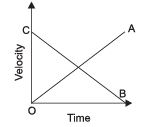OA − shows uniform acceleration.
CB − shows uniform retardation.

Q6. What conclusion do you draw about acceleration of the particle in motion from given velocity-time graph?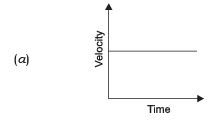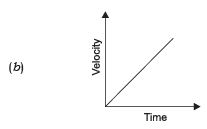Ans. (a) No acceleration
(b) Uniform acceleration

Q7. A car accelerates uniformly from 20 km/h to 35 km/h in 5 s. Calculate
(i) the acceleration and
(ii) the distance covered by the car in that time.
Ans.

u= 20km/h = 5.5m/s

v= 35km/h = 9.72 m/s

t=5s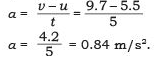(ii) The distance covered by the car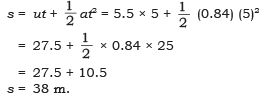Q8. The odometer of a car reads 1800 km at the start of a trip and 2400 km at the end of the trip. If the trip took 10 h. Calculate the average speed of the car in km/h and m/s.
Sol.
Distance covered by the car
s = 2400 − 1800 = 600 km.
Trip time = 10 hr
Average speed = ?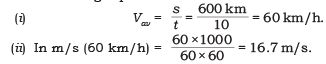The average speed of the car in km/h = 60 km/h and in m/s is 16.7 m/s.

Q9. An object travels 16 m in 4 s and then another 16 m in 3 s. What is the average speed of the object?
Ans.
Total distance travelled by the object = 16 m + 16 m = 32 m

Total time taken = 4s +3s = 7s

∴ Average speed = Total distance travelled/ Total time taken

= 32/7 = 4.57 m/s

∴ The average speed of the object is 4.57 m/s.

Q10. It took 2 s after lightning for the sound of thunder to reach you. How far did the lightning struck?
Ans.
Speed of sound is 346 m/s in air.
Time = 2 s
∴ Distance = ?
s = (d/t)
∴ d = s × t = 346 × 2 = 692 m.
∴ The lightning struck 692 m away.

Q11. Find the type of motion in the following case :
(a) A car travelling along a straight road, changes its speed.
(b) An athlete running a 100 m race.
(c) Moon revolving around the earth.
(d) An ant moving on the floor.
Ans.
(a) Non-uniform motion
(b) Uniform motion
(c) Uniform circular motion
(d) Non-uniform motion

Q12. What conclusion do you draw about acceleration of the particle in motion from given velocity-time graphs.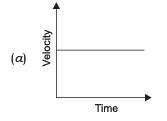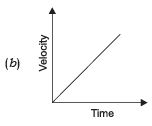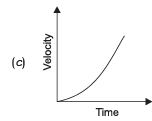Ans. (a) No acceleration (b) Uniform acceleration (c) Non-uniform acceleration

Q13. Derive the equation for velocity-time relation (v = u + at) by graphical method.
Ans.
Let the initial velocity of a body be u = OA = CD.
The final velocity of a body be v = OE = CB
Time t = OC = AD
BC = BD + DC
= BD + OA
∴ v = BD + u
∴ BD = v − u ...(1)

In velocity-time graph, slope gives acceleration.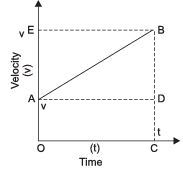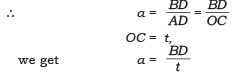∴ BD = at              ...(2)
Substitute (2) in (1) we get.
BD = v − u
at = v − u
∴  v = u + at

Q14. How can we get speed from distance-time graph?
Ans.
Let us assume that an object moves with a uniform speed. The distance-time graph will be a straight line.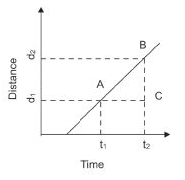let d1 be the distance covered in time t1
Let d2 be the distance covered in time t2
∴ d2 - d1 will be the distance covered in t2-t1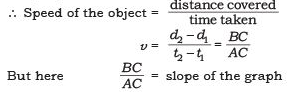Conclusion:

To find the speed from distance-time fraph we can get ots slope which equal to the speed of the object

Q15. Represent a graph that shows the following:
(a) Uniform speed
(b) Non-uniform speed
(c) Stationary object
Ans.
Time is taken on x-axis as it is independent quantity and all dependent quantities are taken along y-axis.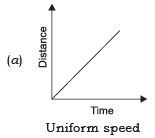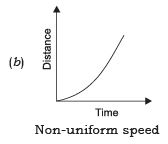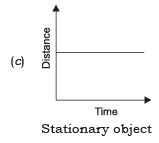Q16. Draw graph to show the following:
(a) Uniform acceleration
(b) Non-uniform acceleration
(c) Uniform motion
Ans. (a)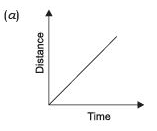Uniform acceleration - A body travels equal distance in equal intervals of time.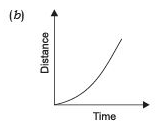Non-uniform acceleration - A body travels unequal distance in equal interval of time.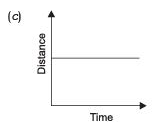Uniform motion or zero accelation - A body moves with constant speed in same line.

Q17. How can you get the distance travelled by on object from its speed-time graph?
Ans.
Suppose an object is moving with a same speed v, the distance covered by this object when it was at (point A)

t1 to t2 is :

AB = t- t1
∵   v = distance/time

∴ distance = v(t- t1)

∴ s = area of rectangle ABCD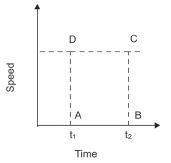∴ To find the distance travelled by a body from its speedtime graph, we need to find the area enclosed by the graph.

Q18. Define acceleration and give its SI unit. When is acceleration of a body negative? Give two examples of situations in which acceleration of the body is negative.
Ans.
The change in velocity per unit time is called acceleration. Its unit is m/s2.
When the velocity of the body decreases it is negative, for e.g., a moving car applies breaks to stop, it is called deceleration. When a body falling due to the gravity, free fall is also considered to be negative –9.8m/s2.

Q19. Distinguish between uniform motion and non- uniform motion.
Ans.
Uniform motion: When a body travels equal distance in equal intervals of time, is called uniform motion.

Non-uniform motion: When a body travels unequal distance in equal intervals of time it is called non-uniform motion.

Q20. The below data shows the speedometer readings of a car. Calculate the acceleration of the car.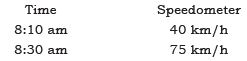Ans.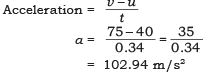Q21. Velocity-time graph for the motion of an object in a straight path is a straight line parallel to the time axis. Comment on any two analysis from such a graph.
Ans.
The nature of the motion is uniform.
The object is not accelerating.

Q22. Draw the shape of the distance-time graph for uniform and non-uniform motion of object.
Ans.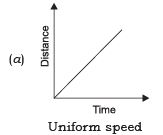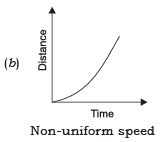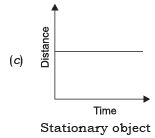Q23. A bus of starting from rest moves with uniform acceleration of 0.5 ms–2 for 2 minutes. Find
(a) the speed acquired.
(b) the distance travelled.
Ans. (a)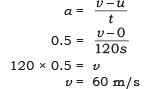(b)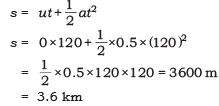Q24. A car accelerates uniformly from 50 km/h to 85 km/h in 10 seconds. Calculate
(a) acceleration in m/s2.
(b) distance covered by the car in metres during the given time of interval.

Ans. (a) a = 85 – 50 = 35 km/h =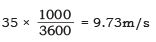a = 9.73m/s

50km/h = 13.89 m/s

85km/h = 23.62 m/s

(b) There are two ways to find the distance covered by the car in t = 10 seconds.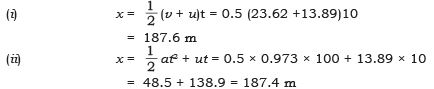Q25. A vehicle decelerates from 68 ms–1 to 16 ms–1 in 8 sec. Calculate
(a) the deceleration and
(b) the distance covered by the car in that time.
Ans. (a)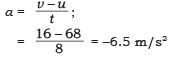(b) Distance covered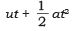= 68 × 8 – 0.5 × 6.5 × 8 × 8 = 544 – 208 = 336 m

Q26. (a) Differentiate between speed and velocity.
(b) When is a body said to have uniform velocity?
(c) How can we describe the position of an object? Illustrate with suitable example.
Ans.
(a) Speed is distance traveled per unit time and velocity is displacement per unit time.
(b) Uniform velocity: when a body travels equal distance in equal intervals of time.
(c) The position of an object is described by the displacement shown by an object from its original position. For e.g., a boy travels in a circular track from a start point and reaches the finish point which is the same point. Hence the displacement is zero.

Q27. Define the term displacement. Is it a vector quantity or a scalar quantity?
Ans.
The distance moved by a body from its mean position is called displacement. It is a vector quantity.

Q28. What is circular motion? Is circular motion an acceleration motion?
Ans.
Uniform circular motion can be described as the motion of an object in a circle at a constant speed. Yes, as an object moves in a circle, it constantly changes its direction.

Q29. A car is travelling with a speed of 90km/h. The driver applied the brakes and retards the car uniformly. The car is stopped in 10sec. Find:
(a) The acceleration of car.
(b) Distance before it stops after applying breaks.
Ans.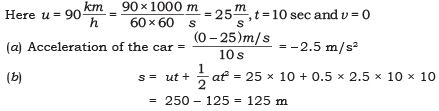Q30. Give two examples of a situation when the displacement can be zero.
Ans.
(a) The displacement can be zero if the start point of the object is same as the end point.
(b) The body reaching the same point after travelling a particular distance. A body in circular motion.

Offer running on EduRev: Apply code STAYHOME200 to get INR 200 off on our premium plan EduRev Infinity!

75 docs|14 tests

,

,

,

,

,

,

,

,

,

,

,

,

,

,

,

,

,

,

,

,

,

,

,

,

,

,

,

;